### Home > CALC > Chapter Ch10 > Lesson 10.3.2 > Problem10-124

10-124.
1. Find the radius and interval of convergence for the following power series. Homework Help ✎

1.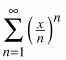2.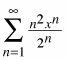3.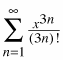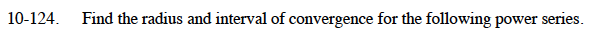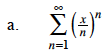$\lim_{n\to\infty}\frac{x^{n+1}/(n+1)^{n+1}}{x^n/n^n}$

$=\lim_{n\to\infty}\frac{x}{n+1}\cdot\Big(\frac{n}{n+1}\Big)^n$

$\lim_{n\to\infty}\frac{xe}{n+1}=?$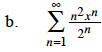$\lim_{n\to\infty}\frac{(n+1)^2x^{n+1}/2^{n+1}}{n^2x^n/2^n}$

$=\lim_{n\to\infty}\frac{x(n+1)^2}{2n^2}=\frac{x}{2}$

$\Big|\frac{x}{2}\Big|\le1$
$\sum_{n=1}^{\infty}\frac{n^2\cdot2^n}{2^n}\text{ and }\sum_{n=1}^{\infty}\frac{n^2\cdot(-2)^n}{2^n}$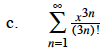$\lim_{n\to\infty}\frac{x^{3n+3}/(3n+3)!}{x^{3n}/(3n)!}$
$\lim_{n\to\infty}\frac{x^3}{(3n+3)(3n+2)(3n+1)}=?$